# Polynomial Class 10 Notes: Chapter 2

## CBSE Class 10 Maths Notes Polynomial:-

Polynomials for class 10 concepts are given in detail. Go through the below article to learn the concept of polynomials such as expressions, degrees, types, graphical representation and so on.

## Algebraic Expressions

An algebraic expression is an expression made up of variables and constants along with mathematical operators.

An algebraic expression is a sum of terms, which are considered to be building blocks for expressions.

A term is a product of variables and constants. A term can be an algebraic expression in itself.
Examples of a term – 3 which is just a constant.
– 2x, which is the product of constant ‘2’ and the variable ‘x’
– 4xy, which is the product of the constant ‘4’ and the variables ‘x’ and ‘y’.
– 5x2y, which is the product of 5, x, x and y.

The constant in each term is referred to as the coefficient.

Example of an algebraic expression: 3x2y+4xy+5x+6 which is the sum of four terms: 3x2y, 4xy, 5x and 6.

An algebraic expression can have any number of terms. The coefficient in each term can be any real number. There can be any number of variables in an algebraic expression. The exponent on the variables, however, must be rational numbers.

### Polynomial

An algebraic expression can have exponents that are rational numbers. However, a polynomial is an algebraic expression in which the exponent on any variable is a whole number.

5x3+3x+1 is an example of a polynomial. It is an algebraic expression as well.

2x+3√x is an algebraic expression, but not a polynomial. – since the exponent on x is 1/2 which is not a whole number.

### Degree of a Polynomial

For a polynomial in one variable – the highest exponent on the variable in a polynomial is the degree of the polynomial.

Example: The degree of the polynomial x2+2x+3 is 2, as the highest power of x in the given expression is x2.

## Types Of Polynomials

Polynomials can be classified based on:
a) Number of terms
b) Degree of the polynomial.

### Types of polynomials based on the number of terms

a) Monomial – A polynomial with just one term. Example: 2x, 6x2, 9xy

b) Binomial – A polynomial with two terms. Example: 4x2+x, 5x+4

a) Trinomial – A polynomial with three terms. Example: x2+3x+4

### Types of Polynomials based on Degree

Linear Polynomial

A polynomial whose degree is one is called a linear polynomial.
For example, 2x+1 is a linear polynomial.

A polynomial of degree two is called a quadratic polynomial.
For example, 3x2+8x+5 is a quadratic polynomial.Cubic Polynomial

A polynomial of degree three is called a cubic polynomial.
For example, 2x3+5x2+9x+15 is a cubic polynomial.

## Graphical Representations

Let us learn here how to represent polynomial equation on the graph.

### Representing Equations on a Graph

Any equation can be represented as a graph on the Cartesian plane, where each point on the graph represents the x and y coordinates of the point that satisfies the equation. An equation can be seen as a constraint placed on the x and y coordinates of a point, and any point that satisfies that constraint will lie on the curve

For example, the equation y = x, on a graph, will be a straight line that joins all the points which have their x coordinate equal to their y coordinate. Example – (1,1), (2,2) and so on.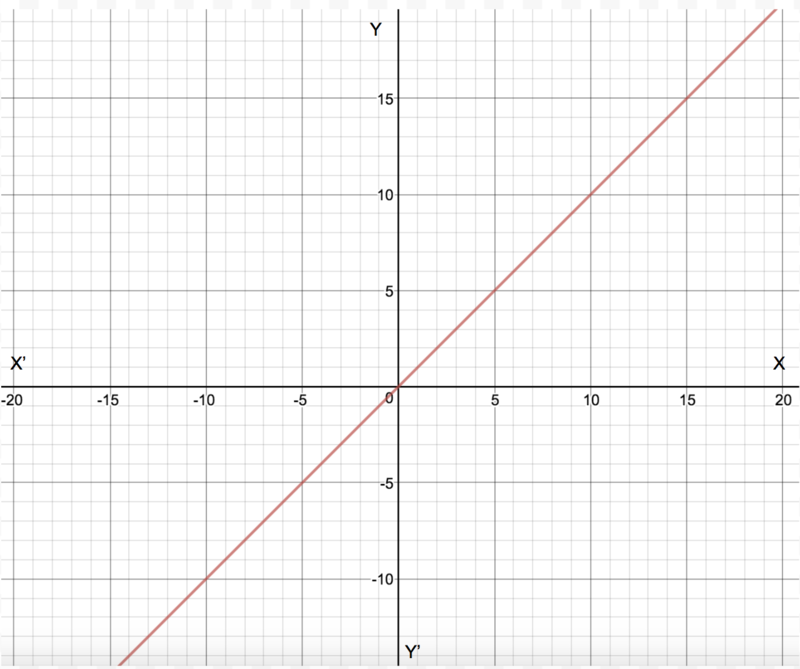### Geometrical Representation of a Linear Polynomial

The graph of a linear polynomial is a straight line. It cuts the X-axis at exactly one point.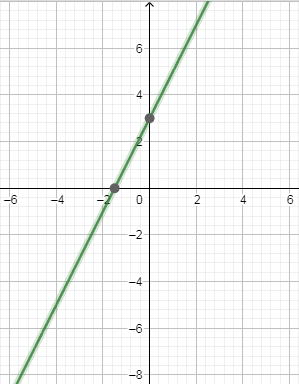### Geometrical Representation of a Quadratic Polynomial

• The graph of a quadratic polynomial is a parabola
• It looks like a U which either opens upwards or opens downwards depending on the value of ‘a’ in ax2+bx+c
• If ‘a’ is positive, then parabola opens upwards and if ‘a’ is negative then it opens downwards
• It can cut the x-axis at 0, 1 or two points
•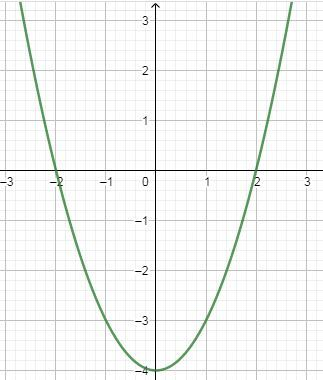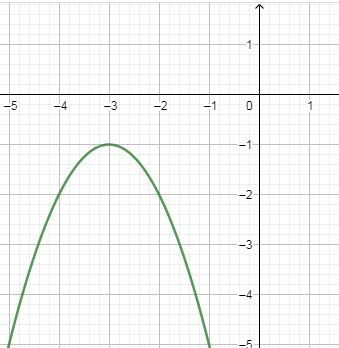### Graph of the polynomial x^n

For a polynomial of the form y=xn where n is a whole number:

• as n increases, the graph becomes steeper or draws closer to the Y-axis
• If n is odd, the graph lies in the first and third quadrants
• If n is even, the graph lies in the first and second quadrants
• The graph of y=−xn is the reflection of the graph of y=xn on the x-axis
•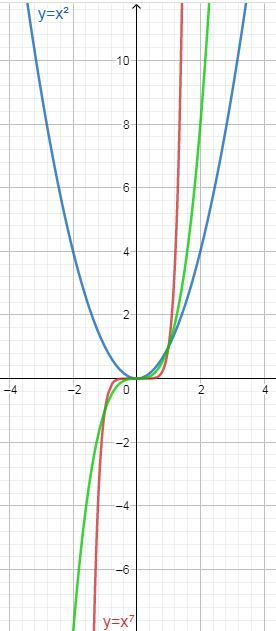## Zeroes of a Polynomial

A zero of a polynomial p(x) is the value of x for which the value of p(x) is 0. If k is a zero of p(x), then p(k)=0.
For example, consider a polynomial p(x)=x2−3x+2.
When x=1, the value of p(x) will be equal to
p(1)=12−3×1+2
=1−3+2
=0

Since p(x)=0 at x=1, we say that 1 is a zero of the polynomial x2−3x+2

### Geometrical Meaning of Zeros of a Polynomial

Geometrically, zeros of a polynomial are the points where its graph cuts the x-axis.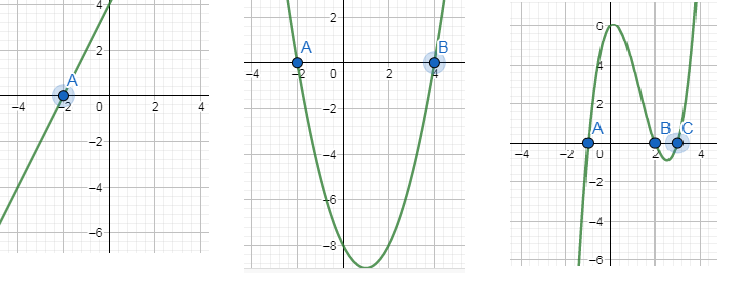Here A, B and C correspond to the zeros of the polynomial represented by the graphs.

### Number of Zeros

In general, a polynomial of degree n has at most n zeros.

1. A linear polynomial has one zero,
2. A quadratic polynomial has at most two zeros.
3. A cubic polynomial has at most 3 zeros.

## Factorisation of Polynomials

Quadratic polynomials can be factorized by splitting the middle term.

For example, consider the polynomial 2x2−5x+3

Splitting the middle term:

The middle term in the polynomial 2x2−5x+3 is -5x. This must be expressed as a sum of two terms such that the product of their coefficients is equal to the product of 2 and 3 (coefficient of x2 and the constant term)

−5 can be expressed as (−2)+(−3), as −2×−3=6=2×3

Thus, 2x2−5x+3=2x2−2x−3x+3

Now, identify the common factors in individual groups

2x2−2x−3x+3=2x(x−1)−3(x−1)

Taking (x−1) as the common factor, this can be expressed as:

2x(x−1)−3(x−1)=(x−1)(2x−3)## Relationship between Zeroes and Coefficients of a Polynomial

If α and β are the roots of a quadratic polynomial ax2+bx+c, then,

α +  β = -b/a

Sum of zeroes = -coefficient of x /coefficient of x2

αβ = c/a

Product of zeroes = constant term / coefficient of x2

For Cubic Polynomial

If α,β and γ are the roots of a cubic polynomial ax3+bx2+cx+d, then

α+β+γ = -b/a

αβ +βγ +γα = c/a

αβγ = -d/a

## Division Algorithm

To divide one polynomial by another, follow the steps given below.

Step 1: arrange the terms of the dividend and the divisor in the decreasing order of their degrees.

Step 2: To obtain the first term of the quotient, divide the highest degree term of the dividend by the highest degree term of the divisor  Then carry out the division process.

Step 3: The remainder from the previous division becomes the dividend for the next step. Repeat this process until the degree of the remainder is less than the degree of the divisor.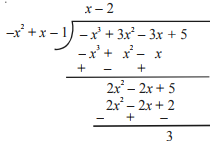## Algebraic Identities

1. (a+b)2=a2+2ab+b2
2. (a−b)2=a2−2ab+b2
3. (x+a)(x+b)=x2+(a+b)x+ab
4. a2−b2=(a+b)(a−b)
5. a3−b3=(a−b)(a2+ab+b2)
6. a3+b3=(a+b)(a2−ab+b2)
7. (a+b)3=a3+3a2b+3ab2+b3
8. (a−b)3=a3−3a2b+3ab2−b31. B Hema Shalini

it was very useful

2. Sushi

This notes helped me a lot.

Very helpful and best material ever!!!!!!!!!!!1111

4. Sandy